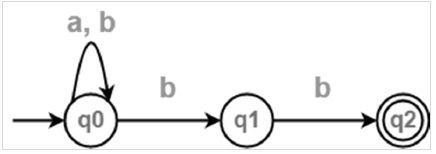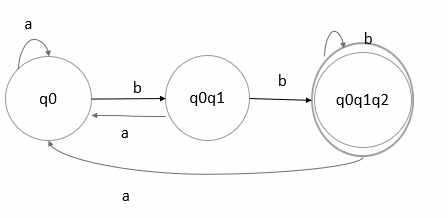# Explain with an example how to convert NFA to DFA.

## Problem

Convert the following Non-Deterministic Finite Automata (NFA) to Deterministic Finite Automata (DFA).

## Solution

The transition diagram is as follows −The transition table of NFA is as follows −

Stateab
->q0q0q0,q1
q1-*q2
*q2--

The DFA table cannot have multiple states. So, make q0q1 as a single state.

Let’s convert the given NFA to DFA by considering two states as a single state.

The transition table of DFA is as follows −

Stateab
->q0q0[q0,q1]
[q0q1][q0][q0q1q2]
*[q0q1q2][q0][q0q1q2]

In the above transition table, q2 is the final state. Wherever, q2 is present that becomes the final state.Note

• After conversion the number of states in the final DFA may or may not be the same as in NFA.
• The maximum number of states present in DFA may be 2pow (number of states in NFA)
• The relationship between number of states in NFA and DFA is: 1<=n<=2m

Where n= number of states in DFA

m= number of states in NFA

• The final DFA all states that contain the final states of NFA are treated as final states.